## Title

Forces and their effects
Quick revise

Mass and weight

Mass is measured in kilograms. An object has the same mass on the Earth, on the Moon, or far out in deep space. Weight is a force and is measured in newtons. Weight is due to gravity attracting the mass towards the centre of the Earth. In deep space there is no gravity and the mass has no weight. On the Moon, which has less mass than the Earth, the gravitational attraction is less, so objects will have less weight than they have on the Earth (only one sixth).

Remember: Weight (N) = mass (kg) x gravitational field strength (N/kg)

Gravitational field strength close to the surface of the Earth is assumed to be a constant 10 N/kg.

Objects in free fall have a constant acceleration of 10 m/s2

This video explains the effects of Gravity

The resultant force and balanced forces
Forces have size and direction. On diagrams they are represented by arrows, in the direction the force acts. The length of the arrow represents the size of the force.
When an object has several forces acting on it, the effect is the same as one force in a certain direction. This is called the resultant force. Fig. 9.5 shows how forces can be combined to give a resultant force. If the resultant force is zero the forces on the object are balanced.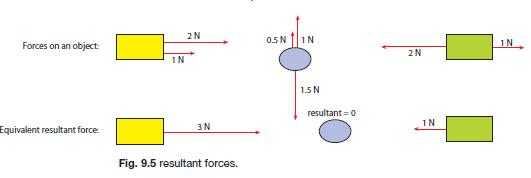A resultant force is needed to change the velocity of an object. If the forces on an object are balanced then it will remain stationary, or, if it is moving, it will continue to move at a steady speed in the same direction. This seems strange because we are used to frictional forces slowing things down.

Remember: If the resultant force on an object is zero, the object will remain stationary or continue to move at a steady speed in the same direction.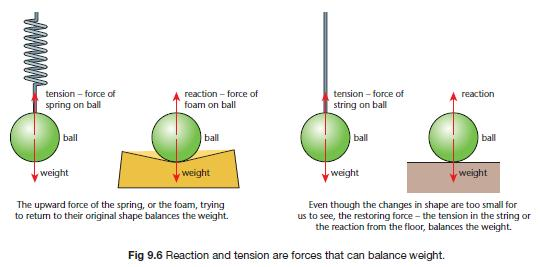This video explains about resultant forces and Terminal Velocity

Resistance to motion – friction and drag
When one object slides over another there is friction, a resistive force between the two surfaces. This arises because, on a microscopic scale, the surfaces are not completely smooth and the high points become stuck together.

Air Resistance

Air resistance (or drag), is a resistive force that acts against objects that are moving through the air. Drag acts on objects moving through any fluid (gas or liquid) – and is larger in liquids.

This video explains about the effects of air resistance

Friction and drag forces:

• always act against the direction of motion
• are zero when there is no movement
• increase as the speed of the object increases.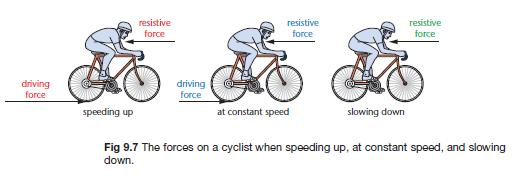When the driving force is larger than the resistive force, the cyclist speeds up; when they are equal he travels at a steady speed, and when the driving force is less than the resistive force he slows down.

The video below shows how balanced and unbalanced forces effect motion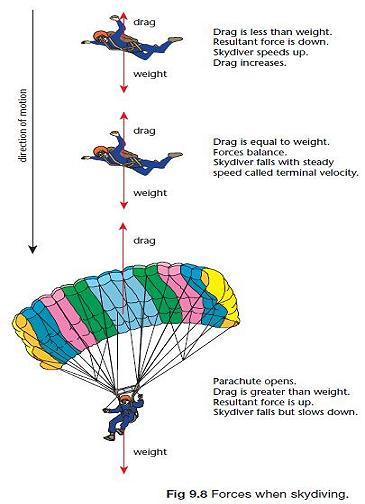When an object falls it can reach a steady speed called terminal velocity where the drag equals the weight

This video explains how forces effect a skydiver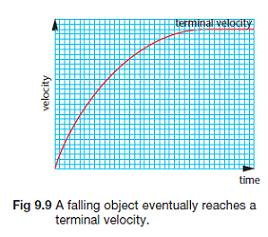When two objects interact, there is always an interaction pair of forces. In  Fig. 9.10, skater A cannot push skater B without skater B pushing skater A.

In an interaction pair of forces, the two forces:
• are always equal in size and opposite in direction
• always act on different objects.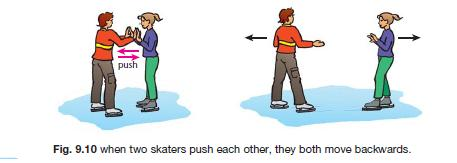Remember: When two objects interact, the forces they exert on each other are equal and opposite and are called action and reaction forces.

Do not confuse these forces, which act on different objects, with balanced forces, which act on the same object.

A rocket and a jet engine make use of this effect. There is an equal and opposite reaction force to the force of the hot exhaust gases pushed out of the back. This reaction force sends the rocket or jet forward. Friction is the force that provides the reaction force you need for walking or for transport using wheels. Fig. 9.11 shows the force of the wheel pushing
back on the road and the reaction – the friction force – of the road pushing forward on the wheel, which sends the wheel forward.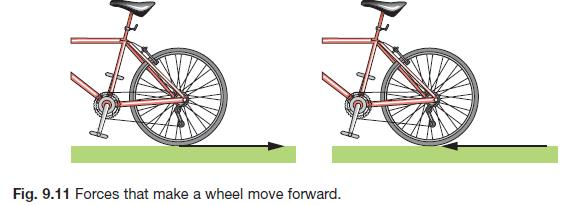When you walk, your foot pushes back on the ground and the ground  pushes forward on your foot.

Imagine walking, or  cycling, on a  frictionless icy  surface. You would  slip back and never  move forward.
Descriptions of what  is pushing/pulling on  what are important  in OCR A.

Force and acceleration

A resultant force on an object causes it to accelerate. The acceleration:

• is in the same direction as the force
• increases as the size of the force increases
• depends on the mass of the object – is smaller for a larger mass.

Remember: For a resultant force on an object with mass m:

Force (N) = mass (kg) x acceleration (m s-2) most GCSE uses m/s2

Stopping distances

The distance that a vehicle travels between the driver noticing a hazard and  when the vehicle stops is called the stopping distance:
stopping distance = thinking distance + braking distance
• Thinking distance is the distance travelled during the driver’s reaction time  – the time between seeing the hazard and applying the brakes.
• Braking distance is the distance travelled while the vehicle is braking.
This diagram shows the shortest stopping distances at different speeds.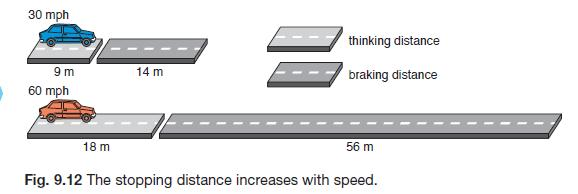When speed doubles,  thinking distance  doubles, and braking  distance is four  times as far.
The stopping distances will also be longer if:
• The driver is tired, or affected by drugs (including alcohol and some
medicines,) or distracted and not concentrating. Reaction time, and
thinking distance are increased.
• The road is wet or icy or the tyres or brakes are in poor condition. The
friction forces will be less, so the braking distance will increase.
• The vehicle is fully loaded with passengers or goods. The extra mass
reduces the deceleration during braking, so the braking distance is increased.
These stopping distances are taken into account when road speed limits are set. Drivers should not drive closer than the thinking distance to the car in front,  allowing them time to react. They should also reduce speed in bad weather to  allow for the increased braking distance.  An anti-lock braking system (ABS) helps to keep the stopping times to a  minimum. If the wheels start to slip because there is not enough friction (making the stopping distance longer), the system disconnects the brakes for a  moment, the wheel grips the road, and the system reapplies the brakes.

This video explains hows to calculate stopping distance

Rate: